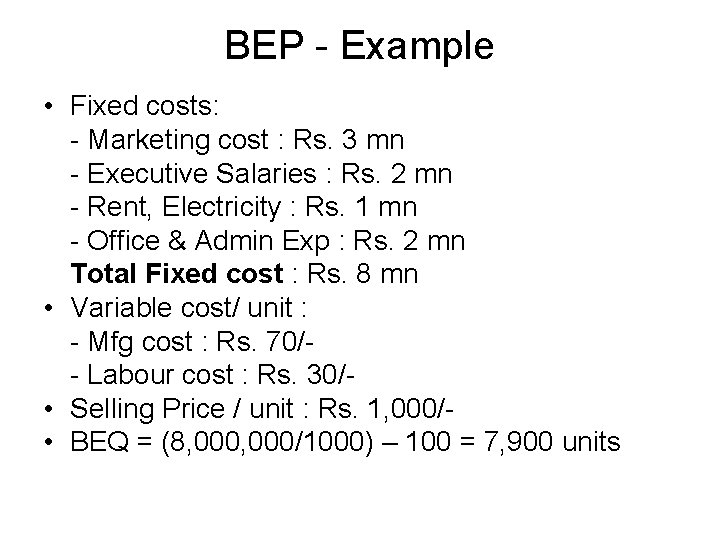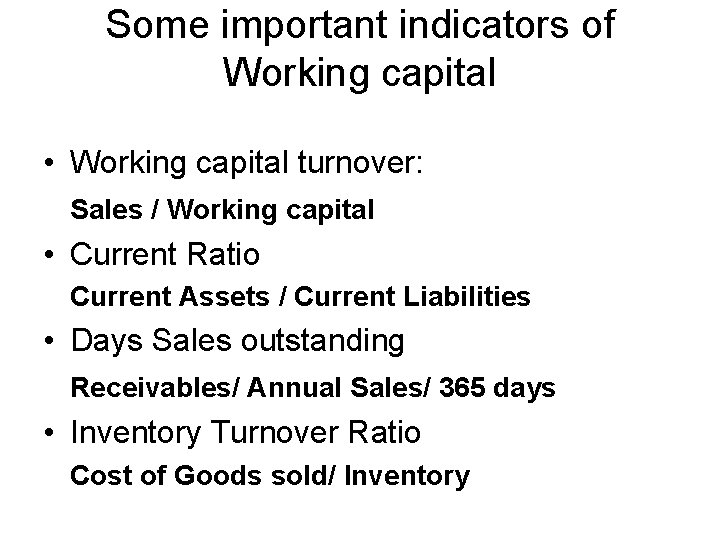# Breakeven Point Sales at which total revenue earned

• Slides: 5Break-even Point • Sales at which total revenue earned equals total costs incurred (TR=TC). • TR = Selling Price x Quantity • TC = Fixed Cost + (Variable Cost/unit x Quantity) • BEQ = FC/SP – VC Break even Analysis • Margin of Safety What is the importance of BEP in a Business? What are the shortcomings of BEP Analysis ?BEP - Example • Fixed costs: - Marketing cost : Rs. 3 mn - Executive Salaries : Rs. 2 mn - Rent, Electricity : Rs. 1 mn - Office & Admin Exp : Rs. 2 mn Total Fixed cost : Rs. 8 mn • Variable cost/ unit : - Mfg cost : Rs. 70/- Labour cost : Rs. 30/ • Selling Price / unit : Rs. 1, 000/ • BEQ = (8, 000/1000) – 100 = 7, 900 unitsCash Flow Management • What is Cash Flow ? • What are Sources and Uses of Cash in a business ? • How important is Cash Flow Management in a business ? • Relationship between Profit/Loss and Cash FlowWorking Capital • What does working capital mean? Working capital = Current Assets – Current Liabilities • What does + ve or – ve working capital indicate ? • How much working capital is adequate for a business ?Some important indicators of Working capital • Working capital turnover: Sales / Working capital • Current Ratio Current Assets / Current Liabilities • Days Sales outstanding Receivables/ Annual Sales/ 365 days • Inventory Turnover Ratio Cost of Goods sold/ Inventory